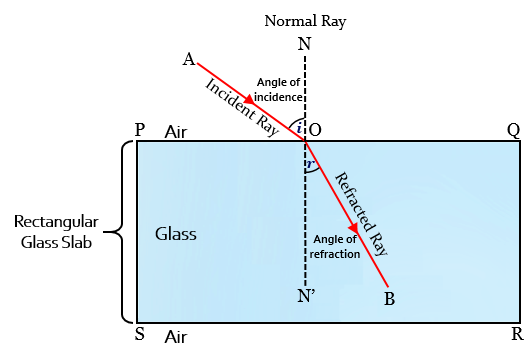# What is meant by the 'angle of incidence' and the 'angle of refraction' for a ray of light? Draw a labelled ray diagram to show the angle of incidence and the angle of refraction for a refracted ray of light.

Angle of incidence:

The angle between the incident ray and normal at the point of incidence is called the angle of incidence.

Angle of refraction:

The angle between the refracted ray and normal at the point of refraction is called the angle of refraction.Updated on: 10-Oct-2022

78 Views